# Digits of age

The product of the digits of Andrew age as 6 years ago and not equal to 0. Andrew age is also the smallest possible age with this two conditions. After how many years will be the product of the digits of Andrew age again the same as today?

Result

n =  18

#### Solution:Leave us a comment of example and its solution (i.e. if it is still somewhat unclear...):

Showing 0 comments:Be the first to comment!## Next similar examples:

1. The largest numberFind the largest integer such that: 1. No figures is not repeat, 2. multiplication of every two digits is odd, 3. addition all digits is odd.
2. MO Z6-6-1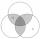Write integers greater than 1 to the blanks in the following figure, so that each darker box was product of the numbers in the neighboring lighter boxes. What number is in the middle box?
3. Prime factorsWrite 98 as product of prime factors
4. Two-digit numberI am a two-digit number less than 20. When I divided by three, then you get the rest 1 and when you divide me by four you get also rest 1. What number am I?
5. Turtles Žofka and Julka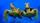Gray wolf kept in his tent turtles Žofka and Julka, which together have 200 years. After 50 years will Julka 2 times older than Žofka. How old are the turtles?
6. Family 8Father is 38 years old, daughter 12, son 14. How many years will father have as many years as his children together?
7. SuzanSusan's age will be after 12 years four times as much as twelve years ago. How old is Susan now?
8. Write decimalsWrite in the decimal system the short and advanced form of these numbers: a) four thousand seventy-nine b) five hundred and one thousand six hundred and ten c) nine million twenty-six
9. Sponsor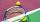The children of the tennis school received 64 white and 48 yellow balls from the sponsor. When asked about how many balls they could take, they were answered: "You have so many that none of you will have more than 10 balls and all will have the same number
10. Lcm of three numbersWhat is the Lcm of 120 15 and 5
11. Unknown number 16My number's tens is three times more then ones My number's ones is twice the number of thousands and my number's hundreds is half the number of of tens. I have two ones. which number am I?
12. SymbolsIf 2*3 = 60 ; 3*4 = 120 and 4*5 = 200, what is 2*5?
13. School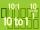Headteacher think whether the distribution of pupils in race in groups of 4,5,6,9 or 10. How many pupils must have at least school at possible options?
14. SickSick Marcel already taken six tablets, which was a quarter of the total number of pills in the pack. How many pills were in the pack?
15. Factors 2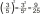A number has 3 identical factors. If 1 of them is 18. Find the number step by step
16. Last pageTwo consecutive sheets dropped out of the book. The sum of the numbers on the sides of the dropped sheets is 154. What is the number of the last page of the dropped sheets?
17. To improve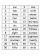To improve her handwriting Paula practices writing the numbers 1 to 200 in words. How many times will she have written the word "one" in all?